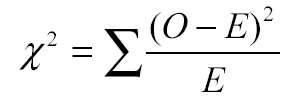Search

Useful Lists

Partners

Online Manuals

Take Web Data Analysis To The Next Level With PHP
By Paul Meagher - 2003-12-22 Page:  1 2 3 4 5 6 7 8 9 10 11 12 13

## Model The Null Hypothesis: The Chi Square Statistic

So far you have summarized the results of your Web poll using a table that reports frequency counts (and percentages) for each response option. To test the null hypothesis (that no difference exists between table cell frequencies), it is easier to compute an overall measure of how much each table cell deviates from the value you would expect under the null hypothesis.

In the case of this beer poll, the expected frequency under the null hypothesis is the following:

 `````` Expected Frequency = Number of Observations / Number of Response Options Expected Frequency = 1000 / 4 Expected Frequency = 250 ``````

To compute an overall measure of how much the responses deviate from the expected frequency per cell, you can sum up all the differences into an overall measure of how much the observed frequencies differ from the expected frequencies: (285 - 250) + (250 - 250) + (215 - 250) + (250 - 250).

If you do this, you find the the expected frequency is 0 because deviations from a mean always sum to 0. To get around this problem, square all the difference scores (hence the square in Chi Square). Finally, to make the score comparable across samples with different numbers of observations (in other words, to standardize it), divide by the expected frequency. So, the formula for the Chi Square statistic looks like this ("O" means "observed frequency" and "E" equals "expected frequency"):

Figure 1. The formula for the Chi Square statisticIf you calculate the Chi Square statistic for the beer poll data, you obtain a value of 9.80. To test your null hypothesis, you want to know the probability of obtaining a value this extreme under the assumption that it is due to random sampling variability. To find this probability, you need to understand what the sampling distribution for Chi Square looks like.

View Take Web Data Analysis To The Next Level With PHP Discussion

Page:  1 2 3 4 5 6 7 8 9 10 11 12 13